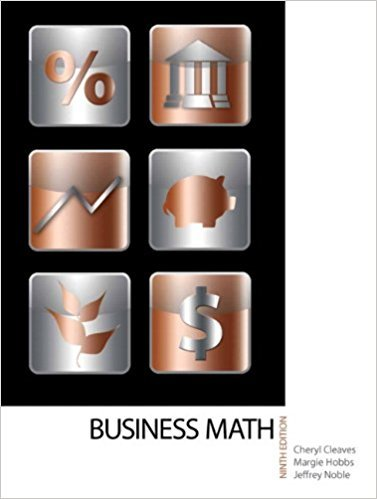×
×

# Multiply. 642 * \$12.98ISBN: 9780135108178 355

## Solution for problem 16 Chapter 3-2

• Textbook Solutions
• 2901 Step-by-step solutions solved by professors and subject experts
• Get 24/7 help from StudySoup virtual teaching assistants4 5 1 423 Reviews
20
1
Problem 16

Multiply. 642 * \$12.98

Step-by-Step Solution:
Step 1 of 3

S343 Section 1.1 Notes- Types of Differential Equations; Exponential Growth/Decay 8-23-16  Exponential Growth and Decay o Rate of change- proportional to amount of “stuff” o Ex. Mouse population growth rate  = =  = 0.5 mice/month (decay...

Step 2 of 3

Step 3 of 3

##### ISBN: 9780135108178

Unlock Textbook Solution